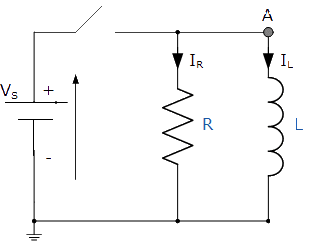# Is it possible to solve for VL in this parallel RL circuit?

• Engineering
bphysics

## Homework Statement

I have the following parallel RC circuit. At time T = 0, the switch will close and the circuit will be connected. We assume that prior to T = 0, the circuit was at a steady-state.I want to calculate VL(t) -- but I am being told that this is not possible for this problem, and would only be possible if there was an additional resistor or inductor (located where the switch is right now).

The professor and other individual who have told me this say that without this additional inductor / resistor, it is not possible to calculate VL(t) as the resistor will be shorted. This makes sense at T = 0, when the inductor will act as a short circuit, and the resistor will in-effect be shorted (as VL(0) = 0).. However, as T increases, the inductor will charge, and the voltage across the inductor will increase, until it acts as an open circuit and all current flows through R.

Therefore, I do not understand why it is not possible to solve for VL(t).

Can anyone provide clarification? I think this might just be miscommunication.

## The Attempt at a Solution

hogrampage
You are confusing inductor with capacitor. A capacitor will charge as T increases, which will eventually act as an open circuit.

On the other hand, an inductor will eventually act like a short circuit (after ~5 time constants).

Mentor
If Vs is an ideal voltage source then it will produce ANY amount of current necessary to maintain its voltage. When the switch closes, node A MUST therefore become equal to Vs, and must remain equal to Vs so long as the switch remains closed.

Normally the voltage across an inductor will go to zero as a circuit heads to steady state, with other circuit components like resistors or capacitors taking up the potential drop. Here it cannot, as Vs wants to maintain the potential and there's nowhere else for a drop to occur. The current through the inductor must therefore head off to infinity to satisfy the equation Vs = L di/dt.

For real components, current will be limited by non-ideal factors like internal resistance, stray inductance, stray capacitance, dielectric breakdown, etc. Something will probably go *BANG*